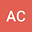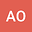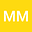Wave-induced steady currents by submerged canopy-oscillatory flow interaction
•••• +1## Abstract

A new analytical model is presented in order to better understand the depth-dependent wave-induced steady current caused by submerged aquatic canopy-oscillatory flow interaction. The analytical model takes into account the wave and canopy properties. The model is developed by determining the dominant terms in the momentum equation by means of dimensional analysis and satisfying the mass conservation. The dimensional analysis reveals that the pressure gradient (due to wave decay) is of the same order of magnitude as the drag force, wave stress and Reynolds stress terms. In addition, the balance between the pressure gradient and mass conservation induces a seaward current above the canopy, and the presence of the pressure gradient in the momentum equation contributes to intensify the skimming flow at the top of the canopy. Finally, given that the model follows a polynomial function it can be easily implemented in large scale models such as phase average models.